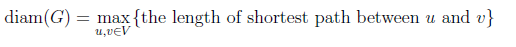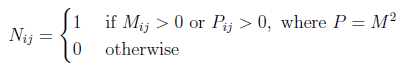Skip to content
Related Articles
GATE | GATE CS 2021 | Set 1 | Question 46
• Last Updated : 24 May, 2021

Let G=(V,E) be an undirected unweighted connected graph. The diameter of G is defined as:Let M be the adjacency matrix of G.

Define graph G2 on the same set of vertices with adjacency matrix N, whereWhich one of the following statements is true?
(A) diam(G2)≤⌈ diam(G)/2⌉
(B) ⌈ diam(G)/2⌉2)< diam(G)
(C) diam(G2) = diam(G)
(D) diam(G)< diam(G2)≤2 diam(G)

Answer: (A)

Explanation: M2 will be the adjacency matrix of the graph H derived from G as follow:

```H(a,b) = 1 iff (there exists a vertex c st. G(a,c) = 1 and G(c,b) = 1)
```

So basically there is an edge between a,b iff there is some vertex c st. there is an edge (a,c) and an edge (c,b) in G.
When we do G∪H in the resulting graph diameter reduces to 0.5 of the original graph

Quiz of this Question

Attention reader! Don’t stop learning now. Learn all GATE CS concepts with Free Live Classes on our youtube channel.

My Personal Notes arrow_drop_up